Courses

Algebra - Functions questions (Part - 2) Notes | Study Quantitative Aptitude for SSC CGL - SSC

SSC: Algebra - Functions questions (Part - 2) Notes | Study Quantitative Aptitude for SSC CGL - SSC

The document Algebra - Functions questions (Part - 2) Notes | Study Quantitative Aptitude for SSC CGL - SSC is a part of the SSC Course Quantitative Aptitude for SSC CGL.
All you need of SSC at this link: SSC

Question 12: The value of f∘g∘h(9) could be, if

f(x) = 1/x
g(x) = 1/(x-2)
h(x) = √x

A. 3

B. 1/3

C. -5

D. None of these

Explanation.

fogoh(9) means f(g(h(9)))
Start by solving h(9) = √9 = 3 and not (3, -3) as square root of a number is always positive.
Taking the value to be 3, g(3) =
1/(3-2) = 1 ⇒ f(1) = 1

The question is "What is value of f∘g∘h(9) could be?"

Hence the answer is "None of these are identical"

Choice D is the correct answer.

Question 13: For this question, assume the following operators:
A*B = A2 - B2

A-B = A/B

A+B = A * B

A/B = A+B

Which of the following expression would yield the result as x subtracted by y?

A. (x*y)-(x+5)

B. (x/y)*(x-y)

C. (x*y)-(x/y)

D. (x+y)*(x-y)

Explanation.

Never solve each expression. Solve by assigning values of x and y
Let x = 6, y = 2 Therefore, required x – y = 4
x*y = 62 – 22 = 32

x-y = 6/2 = 3

x+y = 6 * 2 = 12

x/y = 6+2 = 8

a) 32-12 = 32/12 not equal to 4

b) 8*3 = 82 - 32 = 55 not equal to 4

c) 32 - 8 = 32/8 = 4

d) 12*3 = 122 - 32 not equal to 4

The question is "Which of the following expression would yield the result as x subtracted by y?"

Hence the answer is "(x*y) - (x/y)"

Choice C is the correct answer.

Question 14: Find the domain of: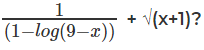A. (-∞,9)

B. [-1,9)

C. [-1,9) excluding 0

D. (-1,9)

Explanation.

In the expression,
9-x > 0
⇒ x < 9
Also, 1-log ( 9-x) ≠ 0
⇒ log (9 – x) ≠ 1
⇒ 9-x ≠ 10
⇒ x ≠ -1
And, x + 1 > 0
⇒ x > -1

The question is "Find the domain of: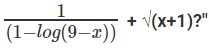Choice D is the correct answer.

Question 15: If [X] – Greatest integer less than or equal to x. Find the value of
[√1] + [√2] + [√3] +……………………………………………………+ [√100]

A. 615

B. 625

C. 5050

D. 505

Explanation.

As can be seen,
Thus, the series becomes:
3 * 1 + 5 * 2 + ……………………………………+ 19 * 9 + 10
∑(2n+1)n + 10 (where, n = 1 to 9)
∑2n2 + ∑n + 10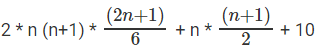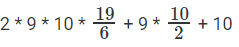⇒ 570 + 45 + 10 625

The question is "Find the value of [√1] + [√2] + [√3] +……………………………………………………+ [√100]?"

Choice B is the correct answer.

Question 16: Find the value of x for which x[x] = 39?

A. 6.244

B. 6.2

C. 6.3

D. 6.5

Explanation.

When x = 7,
x[x] = 7 * 7 = 49
When x = 6,
x[x] = 6 * 6 = 36
Therefore, x must lie in between 6 and 7 ⇒ [x] = 6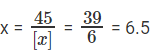The question is "Find the value of x for which x[x] = 39?"

Choice D is the correct answer.

Question 17: Find the value of x for which x[x] = 15?

A. 3.5

B. 5

C. 6.1

D. None of these

Explanation.

Using similar approach as previous question, [x] = 3

x = 15/3 ⇒ x = 5 which is not possible since 3 < x < 4

The question is "Find the value of x for which x[x] = 15?"

Hence the answer is "None of these"

Choice D is the correct answer.

Question 18: If f(x) = 1/g(x), then which of the following is correct?

A. f(f(g(g(f(x))))) = g(f(g(g(g(x)))))

B. f(f(f(g(g(g(f(g(x)))))))) = g(g(g(g(f(g(f(f(x))))))))

C. f(f(g(f(x)))) = g(g(f(g(x))))

D. f(g(f(f(g(f(g(g(x)))))))) = g(g(g(g(f(f(f(f(x))))))))

Explanation.

Given, f(x) = 1/g(x) ⇒ f(x).g(x) = 1 which implies that f(x) and g(x) are essentially inverse of one another.
So, one just has to look for an option which has equal number of fs and gs on both side of the equation.

The question is "If f(x) = 1/g(x), then which of the given is correct?"

Hence the answer is "f(g(f(f(g(f(g(g(x)))))))) = g(g(g(g(f(f(f(f(x))))))))"

Choice D is the correct answer.

Question 19: If f(x) =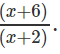Find the value of x for which f(x) = f-1(x)?

A. -3

B. 2

C. Both A and B

D. None of these

Explanation.

f(x) = f-1(x) when f(x) = x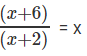x + 6 = x2 + 2x
x2 + x – 6 = 0
(x+3) (x-2) = 0
x = -3, 2

The question is "Find the value of x for which f(x) = f-1(x)?"

Hence the answer is "Both A and B"

Choice C is the correct answer.

Question 20: If f(x) = |x| + |x+3| + |x+6| + ……………………………..+ |x+3t|, where x is an integer and t is a positive integer, find the minimum value of f(x) when t = 6?

A. 63

B. 36

C. 30

D. 25

Explanation.

When t = 3,
f(x) = |x| + |x+3| + |x+6| + ……………………………..+ |x+18|
Minimum value of this function will occur when x = -9 i.e. the middle term is at its minimum which is 0.
Therefore, f(-9) = 9 + 6 + 3 + 0 + 3 + 6 + 9
= 2 * 18
= 36

The question is "find the minimum value of f(x) when t = 6?"

Choice B is the correct answer.

Question 21: In the previous question if t = 7, for how many values of x, f(x) will be minimum?

A. 1

B. 2

C. 4

D. 8

Explanation.

When t = 7,
f(x) = |x| + |x+3| + |x+6| + ……………………………..+ |x+21|
This expression has two middle terms: |x+9|, |x+12|
The value of f(x) will be minimized when the sum of the two middle terms are minimized
=) |x+9|+ |x+12| should be minimum
This happens when -12 ≤ x ≤ -9, Note that for x = -12, -11, -10, -9 the value of the sum of the middle terms = 3.
Therefore, for all 4 values of x, f(x) will have minimum value.

The question is "In the previous question if t = 7, for how many values of x, f(x) will be minimum?"

Choice C is the correct answer.

Question 22If f(x2 – 1) = x4 – 7x2 + k1 and f(x3 – 2) = x6 – 9x3 + k2 then the value of (k2 – k1) is

A. 6

B. 7

C. 8

D. 9

E. None of the above

Explanation.
Given Data

f(x2 – 1) = x4 – 7x2 + k1

f(x3 – 2) = x6 – 9x3 + k2

Approach and Solution

When x2 = 1, f(x2 – 1) = f(1 - 1) = f(0) =(1)2 - 7(1) + k1
f(0) = - 6 + k1 ..........(1)
Essentially, we have replaced all x2 with 1.

When x3 = 2,f(x3 – 2) = f(2 - 2) = f(0) =(2)2 - 9(2) + k2
f(0) = - 14 + k2 ..........(2)
Essentially, we have replaced all x3 with 2.

Equating f(0) in equations (1) and (2)
(-6 + k1) = (-14 + k2)
or k2 - k1 = 8

Question 23Which of the following is not an odd function?

(1) f(x) = -x3

(2) f(x) = x5

(3) f(x) = x2 – x

(4) f(x) = |x|3

Explanation.

An odd function is a function whose value reverses in sign for a reversal in sign of its argument. i.e. f(x) = -f(-x).

Except f(x) = |x|3 all other functions mentioned in the choices change values.

The document Algebra - Functions questions (Part - 2) Notes | Study Quantitative Aptitude for SSC CGL - SSC is a part of the SSC Course Quantitative Aptitude for SSC CGL.
All you need of SSC at this link: SSCUse Code STAYHOME200 and get INR 200 additional OFF Use Coupon CodeQuantitative Aptitude for SSC CGL

54 videos|52 docs|72 tests

Track your progress, build streaks, highlight & save important lessons and more!

,

,

,

,

,

,

,

,

,

,

,

,

,

,

,

,

,

,

,

,

,

;